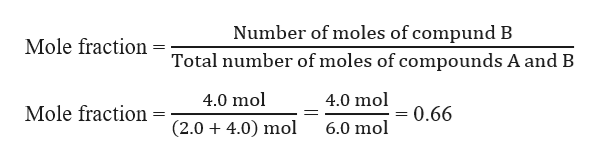# If 2.0 moles of a compound A dissolve in 4.0 moles of another substance B, the mole fraction of B is?

Question
47 views

If 2.0 moles of a compound A dissolve in 4.0 moles of another substance B, the mole fraction of B is?

check_circle

Step 1

Given, Moles of compound A = 2.0

Moles of compound B = 4.0

Mole fraction a component is defined as the ratio of number of moles...help_outlineImage TranscriptioncloseNumber of moles of compund B Mole fraction Total number of moles of compounds A and B 4.0 mol 4.0 mol Mole fraction = 0.66 (2.0 4.0) mol 6.0 mol fullscreen

### Want to see the full answer?

See Solution

#### Want to see this answer and more?

Solutions are written by subject experts who are available 24/7. Questions are typically answered within 1 hour.*

See Solution
*Response times may vary by subject and question.
Tagged in

### General Chemistry